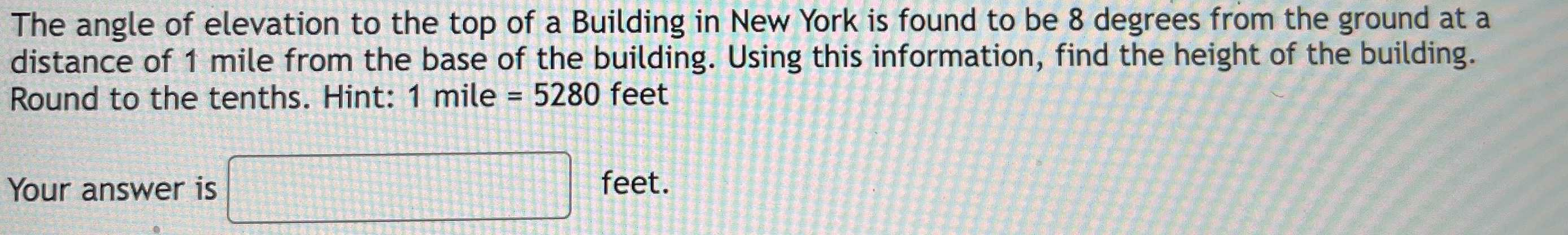### Still have math questions?The angle of elevation to the top of a Building in New York is found to be $$8$$ degrees from the ground at a distance of $$1$$ mile from the base of the building. Using this information, find the height of the building. Round to the tenths. Hint: $$1$$ mile $$= 5280$$ feet Your answer is feet.